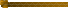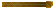prev next# Terminology

What is a decibel (dB)?

The decibel is a relative unit, not an absolute. Decibels are used to compare values of like quantities, usually power and intensity, on a numerical scale. For example:

• An intensity ratio of 10 translates to 10 decibels
• An intensity ratio of 100 translates to a level difference of 20 decibels
• An intensity ratio of 1000 translates to a level difference of 30 decibels

To be meaningful, a decibel needs a reference point. For example:

• In water we use a standard reference sound pressure of 1 microPascal
• In air we use a higher standard of reference of 20 microPascals
• It is essential that sound levels expressed in decibels include the reference pressure
Comparison of Various Noise Sources in the Ocean
Noise Sources Sound Levels
Lightning Strike on Water Surface 260 dB (approximately)
Seafloor Volcanic Eruption 255 dB (approximately)
Sperm Whale 163–180 dB
Fin Whale 160–186 dB
Humpback Whale 144–174 dB